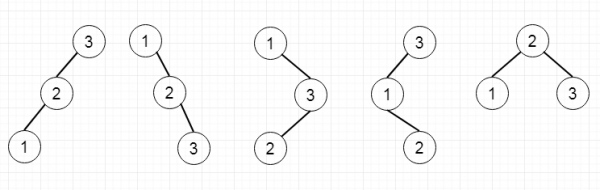# Unique Binary Search Trees in C++

Suppose we have an integer n, we have to count all structurally unique binary search trees that store values from 1 to n. So if the input is 3, then the output will be 5, as the trees will be –To solve this, we will follow these steps –

• create one array of size n + 1
• dp := 1
• for i := 1 to n
• for j := 0 to i – 1
• dp[i] := dp[i] + (dp[i – 1 – j] * dp[j])
• return dp[n]

## Example(C++)

Let us see the following implementation to get a better understanding −

Live Demo

#include <bits/stdc++.h>
using namespace std;
class Solution {
public:
int numTrees(int n) {
vector <int> dp(n+1);
dp = 1;
for(int i =1;i<=n;i++){
for(int j = 0;j<i;j++){
//cout << j << " " << i-1-j << " " << j << endl;
dp[i] += (dp[i-1-j] * dp[j]);
}
}
return dp[n];
}
};
main(){
Solution ob;
cout << ob.numTrees(4);
}

## Input

4

## Output

14